﻿ 散热强化型覆晶球栅数组封装组合体的散热性能研究 On the Thermal Performance of a Thermally Enhanced FC-PBGA Assembly

Applied Physics
Vol.08 No.01(2018), Article ID:23643,12 pages
10.12677/APP.2018.81009

On the Thermal Performance of a Thermally Enhanced FC-PBGA Assembly

J. Y. You, G. H. Wu*

Department of Mechanical Engineering, National Cheng-Kung University, Tainan Taiwan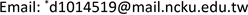Received: Jan. 8th, 2018; accepted: Jan. 24th, 2018; published: Jan. 31st, 2018ABSTRACT

In this paper, three-dimensional finite element analysis using the commercial ANSYS software is performed to study the thermal performance of a thermally enhanced FC-PBGA (Flip-Chip Plastic Ball Grid Array) assembly in both natural and forced convection environments. The thermally enhanced FC-PBGA assembly is a basic FC-PBGA package that is over molded with molding compound, after which an aluminum heat spreader is adhered to the top of the molding compound and subsequently mounted on a PCB (Printed Circuit Board). The thermal behavior of the assembly is presented. Thermal performance is analyzed by considering various design parameters of the aluminum heat spreader, molding compound and adhesive.1. 引言

2. 有限元素仿真

2.1. 覆晶球栅数组组合体描述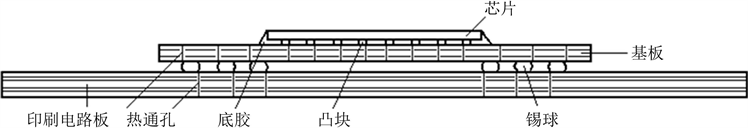Figure 1. Type 1 assembly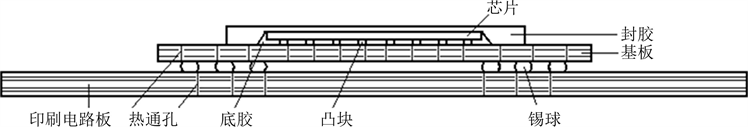Figure 2. Type 2 assembly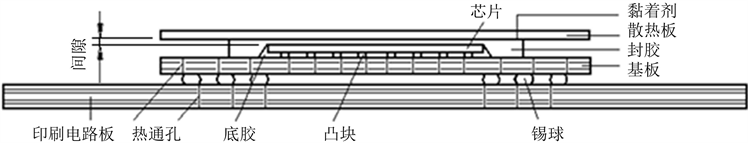Figure 3. Type 3 assembly

2.2. 控制方程式与边界条件

$\frac{\partial }{\partial x}\left({k}_{x}\frac{\partial T}{\partial x}\right)+\frac{\partial }{\partial y}\left({k}_{y}\frac{\partial T}{\partial y}\right)+\frac{\partial }{\partial z}\left({k}_{z}\frac{\partial T}{\partial z}\right)+\stackrel{˙}{g}=0$ (1)

$q=\left({h}_{c}+{h}_{r}\right)\left({T}_{s}-{T}_{{}_{\infty }}\right)$ (2)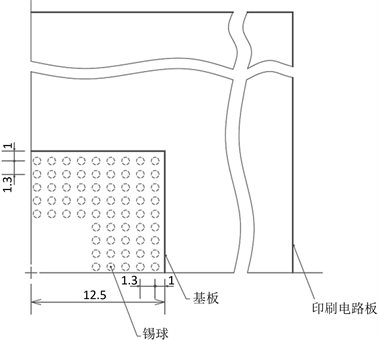Figure 4. Location of solder ballsTable 1. Assembly part dimensions and material conductivities 

${h}_{c}=3.786\sqrt{V/L}$ (3)

${h}_{c}=4.37\sqrt{V/L}$ (4)

${h}_{c}=f{\left(\frac{\Delta {T}_{s-a}}{{L}_{ch}}\right)}^{n}$ (5)

${h}_{r}=Bfe\left({T}_{s}+{T}_{\infty }\right)\left({T}_{s}{}^{2}+{T}_{\infty }{}^{2}\right)$ (6)

2.3. 网格收敛分析

2.4. 热阻

${R}_{ja}=\frac{\left({T}_{j}-{T}_{\infty }\right)}{P}$ (7)

3. 结果与讨论

3.1. 温度分布

FC-PBGA基本型组合体(即第一型组合体)于自然对流环境中的温度分布如图6所示，最高温度发生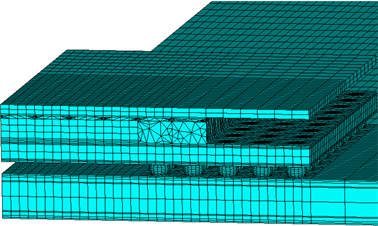Figure 5. Finite element mesh used in simulations for Type 3 assembly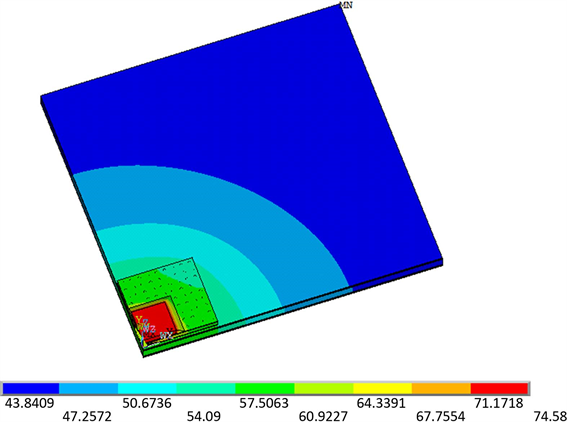Figure 6. Temperature contours for the basic FC-PBGA assembly in natural convection environment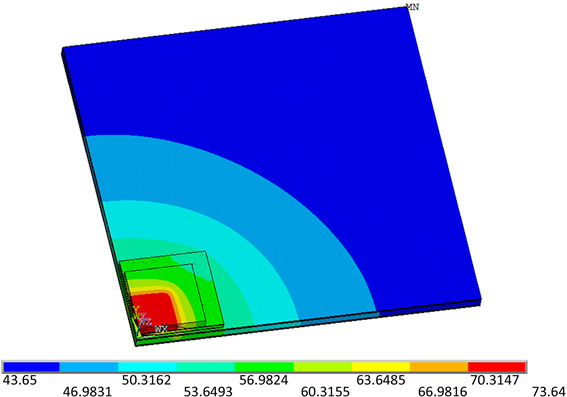Figure 7. Temperature contours for the type 2 assembly in natural convection environment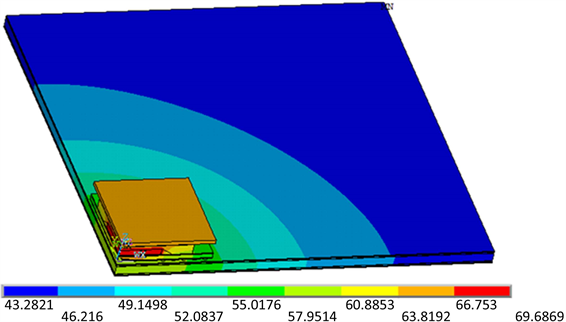Figure 8. Temperature contours for the type 3 assembly in natural convection environment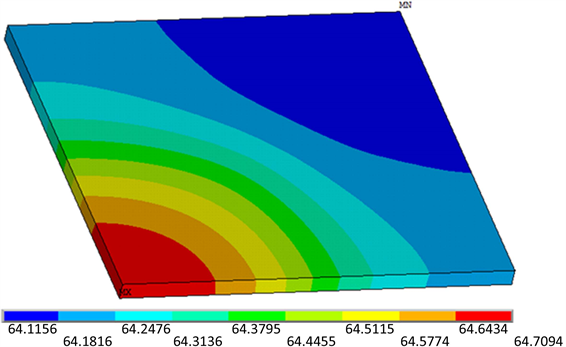Figure 9. Temperature distribution for the heat spreader in natural convection environment

3.2. 设计参数的影响

3.2.1. 铝质散热板的影响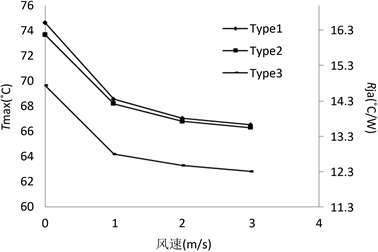Figure 10. Effect of airflow speed on the highest assembly temperature and thermal resistance of the three different types of FC-PBGA assembly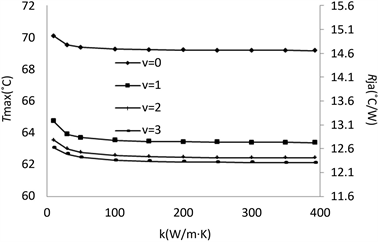Figure 11. Effect of Heater spreader thermal conductivity on the highest assembly temperature and thermal resistance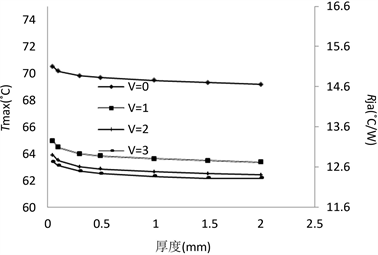Figure 12. Effect of Heater spreader thickness on the highest assembly temperature and thermal resistance

3.2.2. 封胶设计参数的影响

3.2.3. 黏着剂的影响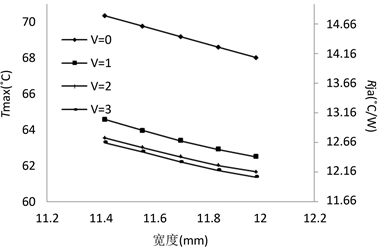Figure 13. Effect of Heater spreader width on the highest assembly temperature and thermal resistance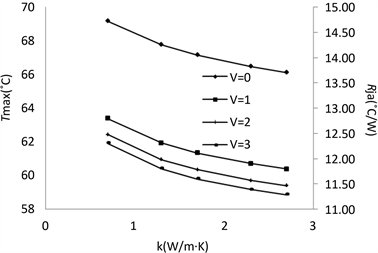Figure 14. Effect of molding compound thermal conductivity on the highest assembly temperature and thermal resistance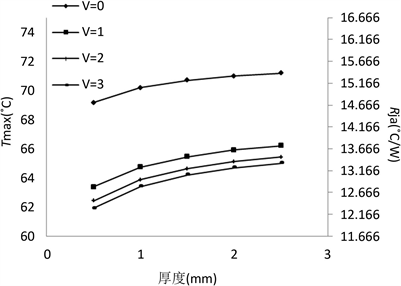Figure 15. Effect of molding compound gap on the highest assembly temperature and thermal resistance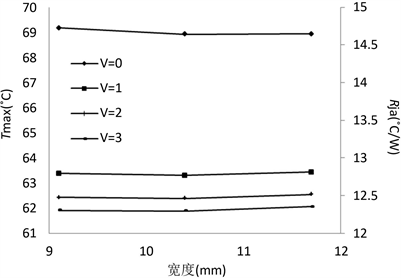Figure 16. Effect of molding compound width on the highest assembly temperature and thermal resistance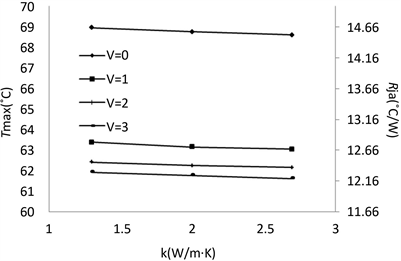Figure 17. Effect of glue thermal conductivity on the highest assembly temperature and thermal resistance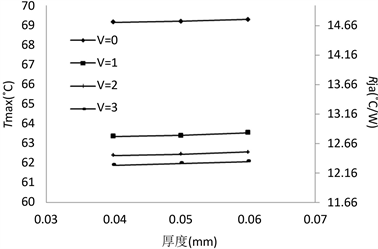Figure 18. Effect of glue thickness on the highest assembly temperature and thermal resistance

4. 结论

1) 第一型、第二型与第三型组合体于自然对流与强制对流环境中最高温度都发生在芯片上。

2) 铝质散热板宽度的影响比厚度的影响显着，这是因为增加宽度可以有效地增加散热板的冷却面积。散热板厚度太大(>2 mm)，会造成材料的浪费而且无法有效地增加组合体的散热。散热板厚度太小(< 0.05 mm)，散热板对组合体散热的帮助会不显着。散热板的导热系数有助于组合体的散热，但是导热系数大于 $50\text{\hspace{0.17em}}\text{W}/\text{m}\cdot \text{K}$ ，增加导热系数对组合体散热只有很小的影响，所以将铝质(导热系数= $226\text{\hspace{0.17em}}\text{W}/\text{m}\cdot \text{K}$ )散热板换成铜质(导热系数= $398\text{\hspace{0.17em}}\text{W}/\text{m}\cdot \text{K}$ )散热板是不必要的。

3) 研究结果发现如果减少封胶的间隙或增加封胶的导热系数有助于组合体之散热，因为二者都会降低热传路径的热阻。封胶宽度对组合体散热性能的影响很小。封胶间隙的影响远大于宽度的影响。

4) 黏着剂对组合体散热性能的影响很小。但是，大一点的导热系数，小一点的厚度有助于组合体散热性能的提升。

On the Thermal Performance of a Thermally Enhanced FC-PBGA Assembly[J]. 应用物理, 2018, 08(01): 69-80. http://dx.doi.org/10.12677/APP.2018.81009

1. 1. Wu, G.H., Ju, S.H. and Tsein, T.C. (2005) Three-Dimensional Finite Element Analysis of Thermomechanical Behavior in Flip-Chip Packages under Temperature Cycling Conditions. Journal of Reinforced Plastics and Composites, 24, 1895-1907. https://doi.org/10.1177/0731684405054333

2. 2. Joiner, B. and Montes de Oca, T. (2002) Thermal Performance of Flip Chip Ball Grid Array Packages. Eighteenth Annual IEEE Semiconductor Thermal Measurement and Management Symposium, 50-56. https://doi.org/10.1109/STHERM.2002.991345

3. 3. Lu, X.N., Shi, T.L. and Liao, G.L. (2008) Thermal Conduction Analysis and Characterization of Solder Bumps in Flip Chip Package. Sciencedirect Applied Thermal Engineering, 36, 181-187. https://doi.org/10.1016/j.applthermaleng.2011.12.028

4. 4. Lee, T.-Y. (2000) An Investigation of Thermal Enhancement on Flip Chip Plastic BGA Packages Using CFD Tool. IEEE Transactions on Components and Packaging Technologies, 23, 481-489. https://doi.org/10.1109/6144.868847

5. 5. Shim, K.W. and Lo, W.Y. (2012) Solder Fatigue Modeling of Flip-Chip Bumps in Molded Packages. Thirty-First IEEE/CPMT International Electronic Manufacturing Technology Symposium, 109-114.

6. 6. Mertol, A. (1996) Thermal Performance Comparison of High Pin Count Cavity-Up Enhanced Plastic Ball Grid Array (EPBGA) Packages. IEEE Transactions on Components, Packaging and Manufacturing Technology: Part B, 19, 427-443.

7. 7. Ellison, G.N. (1989) Thermal Computations for Electronic Equipment. E. Robert, Krieger Publishing Company, Malabar.

8. 8. Chen, W.-H., Cheng, H.-C. and Shen, H.-A. (2003) An Effective Methodology for Thermal Characterization of Electronic Packaging. IEEE Transactions on Components and Packaging Technologies, 26, 222-232. https://doi.org/10.1109/TCAPT.2002.806180

9. NOTES



*通讯作者。